### Home > INT3 > Chapter 11 > Lesson 11.2.1 > Problem11-57

11-57.
1. Add or subtract the following rational expressions. Be sure to look for factors before trying to determine a common denominator, and simplify your answers, if possible. Homework Help ✎

1.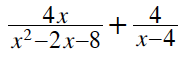2.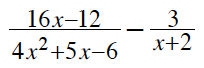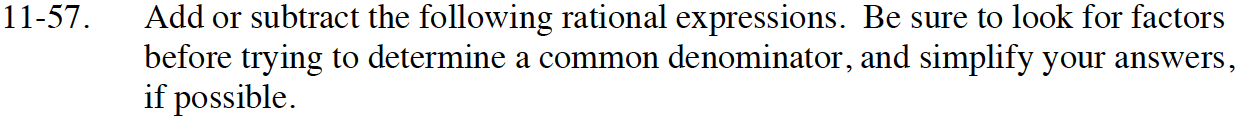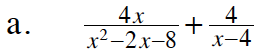This is addition of fractions. What are the steps for adding fractions?

Factor.

$\frac{4x}{(x+2)(x-4)} +\frac{4}{x-4}$

To make common denominators, multiply the second term by the Giant One below.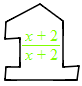$\frac{4x}{(x+2)(x-4)} +\frac{4(x+2)}{(x+2)(x-4)}$

Add the numerators and keep the denominators the same.

$\frac{8x+8}{(x+2)(x-4)}$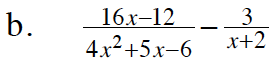Refer to part (a).

$\frac{1}{x+2}$